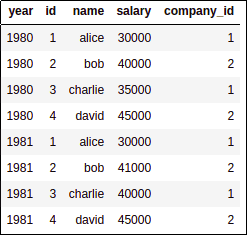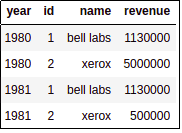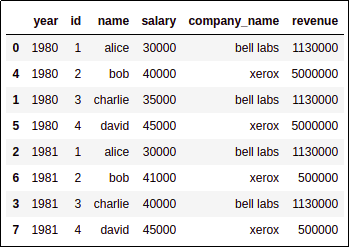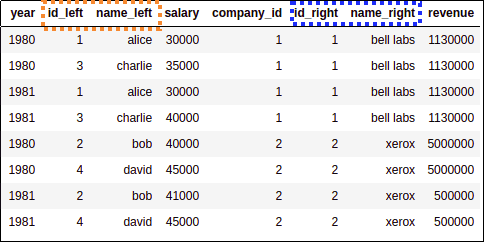Pandas Dataframe: Merge and Join Examples

Last updated:

WIP Alert This is a work in progress. Current information is correct but more content may be added in the future.

View examples on this jupyter notebook

The default is an inner join. Use 'on'='left'|'right'|'outer' to change join types.

pd.merge(
<left_daframe>,
<right_dataframe>,
left_on= <column_on_left_dataframe>,
right_on= <column_on_right_dataframe>)


Join on indices

If the columns you want to join on are Indices, use left_index and right_index:

pd.merge(
<left_daframe>,
<right_dataframe>,
how= <'inner','left','right'>,
left_index=True,
right_index=True)


Join on multiple columns

Joining by multiple columns is useful for dealing with time-stamped data.

Just pass an array of column names to left_on and right_on:

import pandas as pd

df_employees_sal = pd.DataFrame({
'year':[1980,1981,1980,1981,1980,1981,1980,1981],
'id':[1,1,2,2,3,3,4,4],
'name':['alice','alice','bob','bob','charlie','charlie','david','david'],
'salary':[30000,30000,40000,41000,35000,40000,45000,45000],
'company_id':[1,1,2,2,1,1,2,2]})

df_companies_rev = pd.DataFrame({
'year':[1980,1981,1980,1981],
'id':[1,1,2,2],
'name':['bell labs','bell labs','xerox','xerox'],
'revenue':[1130000,1130000,5000000,500000]})

pd.merge(
df_employees_sal,
df_companies_rev,
left_on=['year','company_id'],
right_on=['year','id']
)LEFT: employees and
annual salariesRIGHT: companies and
annual revenueMERGED

pd.merge() vs dataframe.join() vs dataframe.merge()

TL;DR: pd.merge() is the most generic. df.merge() is the same as pd.merge() with an implicit left dataframe. Use df.join() for merging on index columns exclusively.

These are three different ways to do merging/joining dataframes on pandas.

• pd.merge(df_left,df_right): Supports all types of joins

• df_left.join(df_right): Joins df_left and df_right using index columns only.

• df_left.merge(df_right): Same thing as pd.merge(df_left, df_right)

Rename duplicate columns

Pass suffix=(<suffix_for_left>,<suffix_for_right>) to pd.merge():

import pandas as pd

df_employees_sal = pd.DataFrame({
'year':[1980,1981,1980,1981,1980,1981,1980,1981],
'id':[1,1,2,2,3,3,4,4],
'name':['alice','alice','bob','bob','charlie','charlie','david','david'],
'salary':[30000,30000,40000,41000,35000,40000,45000,45000],
'company_id':[1,1,2,2,1,1,2,2]})

df_companies_rev = pd.DataFrame({
'year':[1980,1981,1980,1981],
'id':[1,1,2,2],
'name':['bell labs','bell labs','xerox','xerox'],
'revenue':[1130000,1130000,5000000,500000]})

# suffixes takes a tuple with the suffix values for duplicate columns coming
# from the left and right dataframes, respectively
pd.merge(
df_employees_sal,
df_companies_rev,
left_on=['year','company_id'],
right_on=['year','id'],
suffixes=('_left','_right')
)LEFT: employess and
annual salariesRIGHT: companies and
annual revenueMERGED: Newly named columns are left (orange) and right (blue)
Note that column year was not duplicated,
pandas correctly identified it
was the same in both sides.

merge_asof example

TODO


Approximate match join

I.e. join a row on left_df with a row on right_df where column column_right on right_df is the closest to column_left on left_df, according to a given distance function.

TODO read these


https://stackoverflow.com/questions/13636848/is-it-possible-to-do-fuzzy-match-merge-with-python-pandas

https://stackoverflow.com/questions/34880539/pandas-merging-based-on-a-timestamp-which-do-not-match-exactly

• This looks very promising

https://pandas.pydata.org/pandas-docs/stable/generated/pandas.merge_asof.html

• Maybe I could use this to snap the dates to the nearest month and then do an exact match:

https://pandas.pydata.org/pandas-docs/stable/generated/pandas.DatetimeIndex.snap.html

• merge_closest

https://github.com/RobinFiveWords/merge_closest

• np.piecewise

https://docs.scipy.org/doc/numpy/reference/generated/numpy.piecewise.html

• IntervalIndex

https://stackoverflow.com/a/46526249/436721

Merge on closest date

TODO a particular case of the above?


Merge with date range

TODO a particular case of the above?


Merge by year/month/day of the week

TODO a particular case of the above?